# 一、二叉搜索树

• 每个节点中的值必须大于（或等于）存储在其左侧子树中的任何值。
• 每个节点中的值必须小于（或等于）存储在其右子树中的任何值。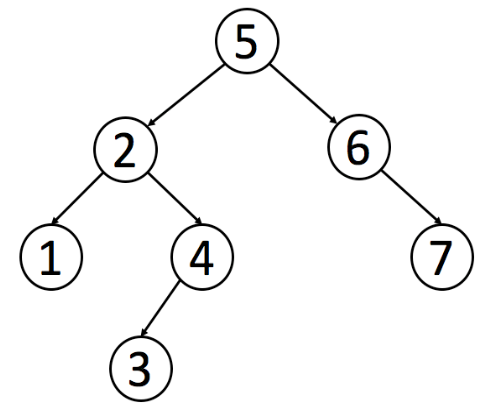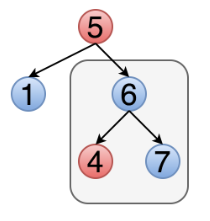## 1、递归

# 递归判断是否为二叉搜索树
class Solution:
def isValidBST(self, root: TreeNode) -> bool:
# 上界初始化为无穷，下界初始化为负无穷
def helper(node, lower = float('-inf'), upper = float('inf')):
if not node:
return True

val = node.val
# 如果当前节点小于下界或大于上界，则不是BST
if val <= lower or val >= upper:
return False
# 考察右子树是否有低于下界的
if not helper(node.right, val, upper):
return False
# 考察左子树是否有高于上界的
if not helper(node.left, lower, val):
return False
# 以上都满足，则是BST
return True

return helper(root)

• 时间复杂度：$O(N)$，每个节点访问了一次
• 空间复杂度：$O(N)$，跟进了整个树

## 2、迭代

# 迭代法，深度优先搜索
class Solution:
def isValidBST(self, root: TreeNode) -> bool:
if not root:
return True
# 初始化栈
stack = [(root, float('-inf'), float('inf')), ]
while stack:
# 出栈
root, lower, upper = stack.pop()
# 如果not root，说明此节点的父节点没有左孩子或右孩子，continue即可
if not root:
continue

val = root.val
# 判断当前节点
if val <= lower or val >= upper:
return False
# 压栈右孩子
stack.append((root.right, val, upper))
# 压栈左孩子
stack.append((root.left, lower, val))
return True

• 时间复杂度：$O(N)$，每个节点访问了一次
• 空间复杂度：$O(N)$，跟进了整个树

## 3、中序遍历

# 中序遍历
class Solution:
def isValidBST(self, root: TreeNode) -> bool:
stack, inorder = [], float('-inf')

while stack or root:
# 到最左边的叶子节点
while root:
stack.append(root)
root = root.left
# 出栈
root = stack.pop()
# 如果它小于它前一个节点的值，则不是BST（因为是中序遍历，是按照递增排的）
if root.val <= inorder:
return False
# 若它大于前一个值，则将当前值赋值给inorder值
inorder = root.val
root = root.right

return True

• 时间复杂度：时间复杂度 : 最坏情况下（树为二叉搜索树或破坏条件的元素是最右叶结点）为$O(N)$
• 空间复杂度：$O(N)$用于存储stack

# 二、基本操作

## 1、在树中搜索某个值

在搜索二叉树中找到某个值并返回改节点，举例如下图所示。我们要找到值为4的节点，从根节点开始，它大于4，因此在左子树找。左子树的根节点小于4，因此再在其右子树找。此时当前节点值为4，返回该节点。此题对于leetcode的第700题。class Solution:
def searchBST(self, root: TreeNode, val: int) -> TreeNode:
# 如果给节点为空，或该节点值等于要找到的值，则返回改节点
if not root or root.val == val:
return root
# 若改节点值大于val，则在左子树找
if root.val > val:
return self.searchBST(root.left,val)
# 否则在右子树找
else:
return self.searchBST(root.right,val)

• 时间复杂度：$O(H)$，其中H是树高。平均时间复杂度：$O(logN)$；最坏时间复杂度：$O(N)$
• 空间复杂度：$O(H)$，递归栈的深度为H。平均情况下深度为$O(logN)$；最坏情况戏曲深度为$O(N)$

class Solution:
def searchBST(self, root: TreeNode, val: int) -> TreeNode:
while root is not None and root.val != val:
root = root.left if val < root.val else root.right
return root

• 时间复杂度：$O(H)$，其中H是树高。平均时间复杂度：$O(logN)$；最坏时间复杂度：$O(N)$
• 空间复杂度：$O(1)$，恒定的额外空间

## 2、插入操作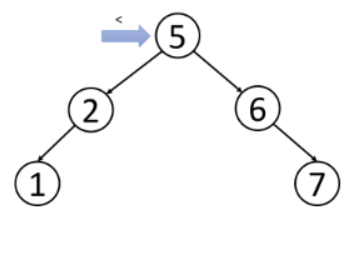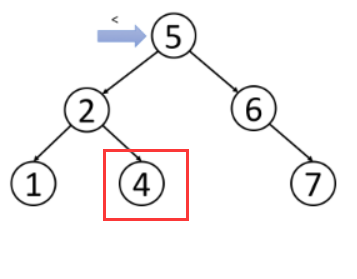class Solution:
def insertIntoBST(self, root: TreeNode, val: int) -> TreeNode:
if not root:
return TreeNode(val)

if val > root.val:
# 在右子树插入
root.right = self.insertIntoBST(root.right, val)
else:
# 在左子树插入
root.left = self.insertIntoBST(root.left, val)
return root

class Solution:
def insertIntoBST(self, root: TreeNode, val: int) -> TreeNode:
node = root
while node:
# 如果val大于当前节点的值，则在右子树插入
if val > node.val:
# 如果没有右孩子，则插入
if not node.right:
node.right = TreeNode(val)
return root
else:
node = node.right
# 如果val大于当前节点的值，则在左子树插入
else:
# 如果没有左孩子，则插入
if not node.left:
node.left = TreeNode(val)
return root
else:
node = node.left
return TreeNode(val)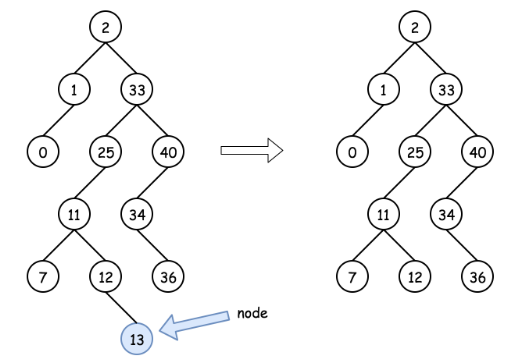• case2：目标节点有右节点，则该节点可以由该节点的后继节点（successor，右子树最左边的节点）进行替代，该后继节点位于右子树中较低的位置。然后可以从后继节点的位置递归向下操作以删除后继节点。如下图所示：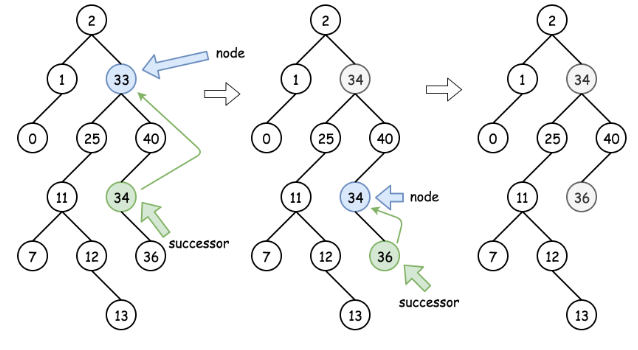图6：删除操作，情况2

• case3：目标节点只有左节点，可以使用它的前驱节点（predecessor，左子树最右边的节点）进行替代，然后再递归的向下删除前驱节点。如下图所示：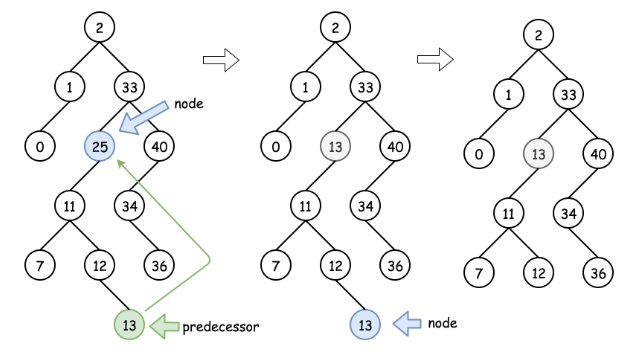图7：删除操作，情况3

class Solution:
def successor(self, root):
# 最左边的节点
root = root.right
while root.left:
root = root.left
return root.val

def predecessor(self, root):
# 最右边的节点
root = root.left
while root.right:
root = root.right
return root.val

def deleteNode(self, root: TreeNode, key: int) -> TreeNode:
if not root:
return None

# 从右子树删除
if key > root.val:
root.right = self.deleteNode(root.right, key)
# 从左子树删除
elif key < root.val:
root.left = self.deleteNode(root.left, key)
# 找到了要删除的节点
else:
# case 1
if not (root.left or root.right):
root = None
# case 2
elif root.right:
root.val = self.successor(root)
root.right = self.deleteNode(root.right, root.val)
# case 3
else:
root.val = self.predecessor(root)
root.left = self.deleteNode(root.left, root.val)

return root

• 时间复杂度：时间复杂度：${O}(\log N)$。在算法的执行过程中，我们一直在树上向左或向右移动。首先先用$O(H_{1})$的时间找到要删除的节点，$H_{1}$是从根节点到要删除节点的高度。然后删除节点需要 ${O}(H_2)$的时间，$H_{2}$指的是从要删除节点到替换节点的高度。由于 ${O}(H_1 + H_2) = {O}(H)$，$H$ 值得是树的高度，若树是一个平衡树则 $H = \log N$。
• 空间复杂度：空间复杂度：$O(H)$，递归时堆栈使用的空间，HH 是树的高度

# 三、例题

class Solution:
def lowestCommonAncestor(self, root: 'TreeNode', p: 'TreeNode', q: 'TreeNode') -> 'TreeNode':
# 递归
# 当p、q的值都小于root时，要在左子树找
if p.val < root.val and q.val < root.val:
return self.lowestCommonAncestor(root.left, p, q)
# 当p、q的值都大于root时，要在右子树找
if p.val > root.val and q.val > root.val:
return self.lowestCommonAncestor(root.right, p, q)
# 否则p或q分列左右子树，root即为最近公共祖先
return root

class Solution:
def lowestCommonAncestor(self, root: 'TreeNode', p: 'TreeNode', q: 'TreeNode') -> 'TreeNode':
# 非递归
while root:
if p.val < root.val and q.val < root.val:
root = root.left
elif p.val > root.val and q.val > root.val:
root = root.right
else:
return root